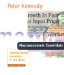# 9.4 The Rules-versus-Discretion Debate

 N3. A bond due to mature and pay \$1,000 in one year's time has a coupon of \$85 and a current price of \$1,025. What is the interest rate?
 N4. Suppose the interest rate is 8 percent, and a bond with an annual coupon of \$75 matures in one year's time, paying its face value of \$1,000. What is this bond's current price?
 N5. Suppose the current interest rate is 6 percent. What price should one expect to pay for a \$1,000 treasury bill, due to mature in six months, that had originally been sold when the interest rate was 7 percent?
 N6. Consider a bond with a face value of \$1,000, due to mature in one year's time. Its current price is \$1,035, and the current interest rate is 5.8 percent. What must its coupon be?
 N7. Suppose "the" multiplier is 3, the income multiplier with respect to the money supply is 4, the money multiplier is 5, and when the central bank buys \$4 billion bonds on the open market during a recession the interest rate falls by one percentage point. Suppose that to fight a recession monetary policy is undertaken to lower the interest rate by one half of a percentage point. What should happen to the income level?
 *N8. Suppose the impact on the interest rate of a \$3 increase in government spending can be eliminated by a \$2 increase in the money supply. If the income multiplier with respect to government spending is 4 and the income multiplier with respect to the money supply is 3, what mix of monetary and fiscal policy is required to increase income by \$9,000 without changing the interest rate?
 N9. In appendix 10.1 information is listed on two notes and a bond that all mature at the same time. Explain why their prices are so different.
 N10. Consider a bond with face value \$1,000, coupon \$100, and price \$927.90, due to mature in five years. It's actual yield to maturity is 12 percent, as reported in section 10.2. What yield to maturity does the shortcut method given in appendix 10.1 produce for this bond?
 N11. Consider a bond with face value \$1,000, coupon \$100, and price \$1,079.85, due to mature in five years. It's actual yield to maturity is 8 percent, as reported in section 10.2. What yield to maturity does the shortcut method given in appendix 10.1 produce for this bond?
 A bond is a formal contract between the issuer of the bond (the borrower) and the owner of the bond (the lender). The issuer promises to pay the face value, or par value, of the bond at a specified future date, the maturity date, as well as make periodic coupon payments until that maturity date. Face values are typically in large round numbers such as \$1,000 or \$10,000, but by tradition bond prices are quoted in percentages.Macroeconomic Essentials - 2nd Edition: Understanding Economics in the News
ISBN: 0262611503
EAN: 2147483647
Year: 2004
Pages: 152

Similar book on Amazon